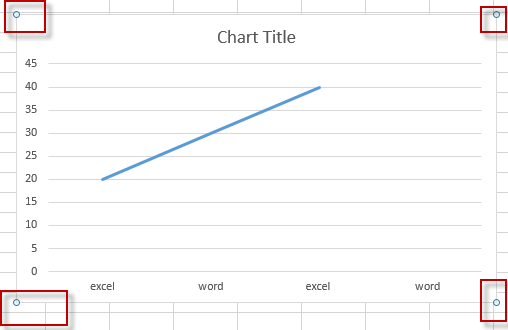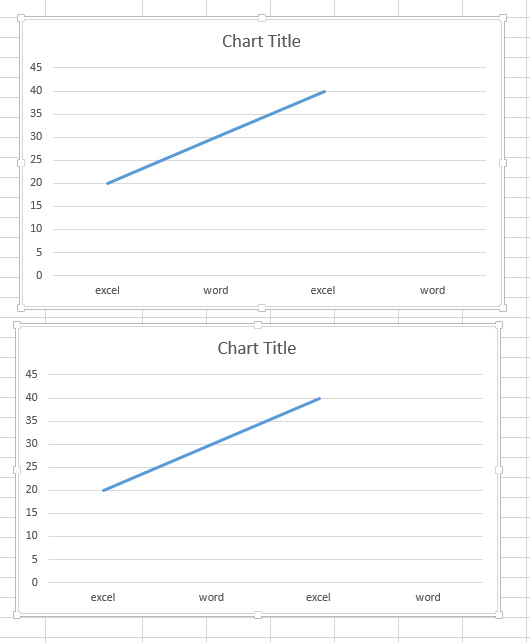# Move Chart with Arrow Keys

This post will guide you how to move chart with arrow keys in your keyboard in excel. How do I move a chart with the arrow keys in excel. You can use the arrow keys (left, down, up, down keys) to move images and shapes in the chart easily, but you are unable to move the chart with arrow keys. How do I move chart to top of sheet with arrow keys in excel.

## Move Chart with Arrow Keys

If you want to move chart with arrow keys (up, down, left ,right) quickly, just do the following steps:

#1 Hold the Ctrl key in your keyboard, and then left click on the chart. Circles will appear on each corner of the current chart.#2 try to move chart with arrow keys on the sheet.

If you want to move multiple charts with arrow keys, just hold the Ctrl key and then select two or more charts using left click by mouse.Related Posts

If Cell is This Value or That Value

IF function is frequently used in Excel worksheet to return you expect “true value” or “false value” based on the result of logical test. If you want to see if a cell is A or B, and if one of ...

If Value is Greater Than A Certain Value

IF function is frequently used in Excel worksheet to return you expect “true value” or “false value” based on the logical test result. If you want to see if a value in one cell is greater than a specific value, ...

If Cell is Not Blank

IF function is frequently used in Excel worksheet to return you expect “true value” or “false value” based on the result of created logical test. If you want to see if a cell is blank or not, and leave some ...

VBA Macro For VLOOKUP From Another Sheet

In the previous post, you should know that how to fix or remove the #N/A error when using VLOOKUP formula to lookup value from another sheet. And this post will show you how to use VBA code to vlookup data ...

If Cell is Blank

IF function is frequently used in Excel worksheet to return you expect “true value” or “false value” based on the result of created logical test. If you want to see if a cell is blank or not, and leave some ...

If Cell Equals Certain Text String

IF function is frequently used in Excel worksheet to return you expect “true value” or “false value” based on the result of created logical test. If you want to see if cell equals a certain text string like “Win”, you ...

If Cell Contains Either Text1 or Text2

IF function is frequently used in Excel worksheet to return “true value” or “false value” based on the logical test result. If you want to see if cell contains certain substring1 like “abc” or substring2 like “def”, and returns true ...

If Cell Contains Certain Text OR Equals Certain Text

IF cell equals certain text IF function is frequently used in Excel worksheet to return “true value” or “false value” based on the logical test result. If you want to test values to see if they equal certain text like ...

VLOOKUP From Another Sheet Not Working

In the previous post, you should know that how to fix or remove the #N/A error when using VLOOKUP formula to lookup value from another sheet. And this post will show you reasons why your VLOOKUP formula is not working ...

If Cell Begins with One of Three Supplied Characters

If you want to test values to see if they begin with some given specific characters like “x”, ”y”, or “z”, you can create a formula with COUNTIF and SUM functions to return results. EXAMPLE You can see “TRUE” or ...

Sidebar